Surge Impedance Loading is a very essential parameter when it comes to the study of power systems as it is used in the prediction of maximum loading capacity of transmission lines.
However before understanding SIL, we first need to have an idea of what is Surge Impedance (Zs). It can be defined in two ways one a simpler one and other a bit rigorous.
Method 1
It is a well known fact that a long transmission lines (> 250 km) have distributed inductance and capacitance as its inherent property. When the line is charged, the capacitance component feeds reactive power to the line while the inductance component absorbs the reactive power. Now if we take the balance of the two reactive powers we arrive at the following equation

Capacitive VAR = Inductive VAR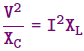Where,
V = Phase voltage
I = Line Current
Xc = Capacitive reactance per phase
XL = Inductive reactance per phase
Upon simplifying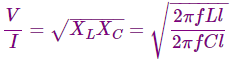Where,
f = Frequency of the system
L = Inductance per unit length of the line
l = Length of the line
Hence we get,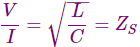This quantity having the dimensions of resistance is the Surge Impedance. It can be considered as a purely resistive load which when connected at the receiving end of the line, the reactive power generated by capacitive reactance will be completely absorbed by inductive reactance of the line.
It is nothing but the Characteristic Impedance (Zc) of a lossless line.

Method 2
From the rigorous solution of a long transmission line we get the following equation for voltage and current at any point on the line at a distance x from the receiving end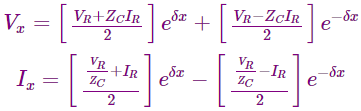Where,
Vx and Ix = Voltage and Current at point x
VR and IR = Voltage and Current at receiving end
Zc = Characteristic Impedance
δ = Propagation Constant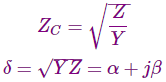Z = Series impedance per unit length per phase
Y = Shunt admittance per unit length per phase
Putting the value of δ in above equation of voltage we get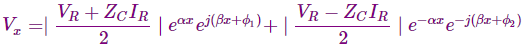Where,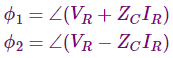We observe that the instantaneous voltage consists of two terms each of which is a function of time and distance. Thus they represent two travelling waves. The first one is the positive exponential part representing a wave travelling towards receiving end and is hence called the incident wave. While the other part with negative exponential represents the reflected wave. At any point along the line, the voltage is the sum of both the waves. The same is true for current waves also.
Now, if suppose the load impedance (ZL) is chosen such that ZL = Zc, and we know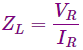Thus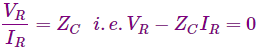and hence the reflected wave vanishes. Such a line is termed as infinite line. It appears to the source that the line has no end because it receives no reflected wave.
Hence, such an impedance which renders the line as infinite line is known as surge impedance.It has a value of about 400 ohms and phase angle varying from 0 to –15 degree for overhead lines and around 40 ohms for underground cables.

The term surge impedance is however used in connection with surges on the transmission line which may be due to lightning or switching, where the line losses can be neglected such that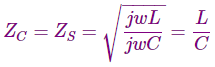Now that we have understood Surge Impedance, we can easily define Surge Impedance Loading.
SIL is defined as the power delivered by a line to a purely resistive load equal in value to the surge impedance of that line. Hence we can write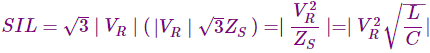The unit of SIL is Watt or MW.
When the line is terminated by surge impedance the receiving end voltage is equal to the sending end voltage and this case is called flat voltage profile. The following figure shows the voltage profile for different loading cases.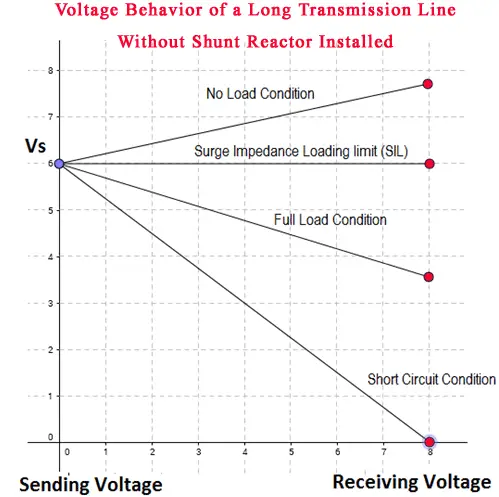It should also be noted that surge impedance and hence SIL is independent of the length of the line. The value of surge impedance will be the same at all the points on the line and hence the voltage.
In case of a Compensated Line, the value of surge impedance will be modified accordingly as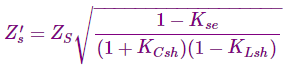Where, Kse = % of series capacitive compensation by Cse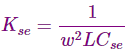KCsh = % of Shunt capacitive compensation by Csh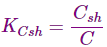Klsh = % of shunt inductive compensation by Lsh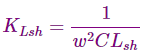The equation for SIL will now use the modified Zs.

Want To Learn Faster? 🎓
Get electrical articles delivered to your inbox every week.
No credit card required—it’s 100% free.